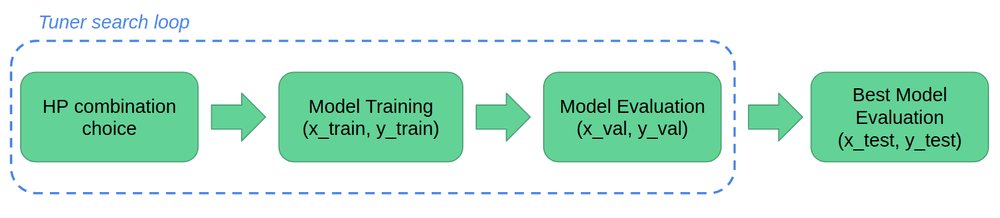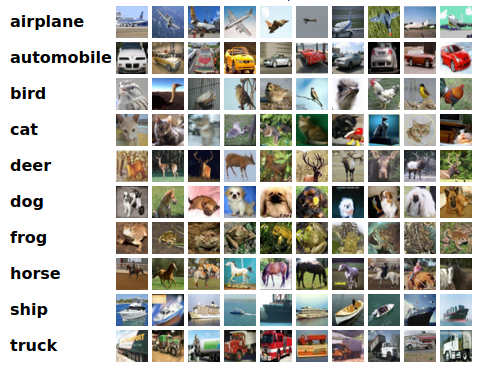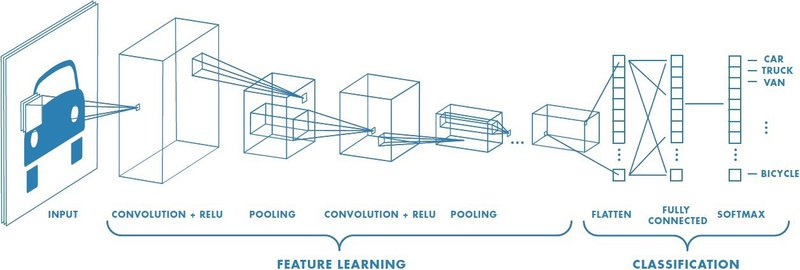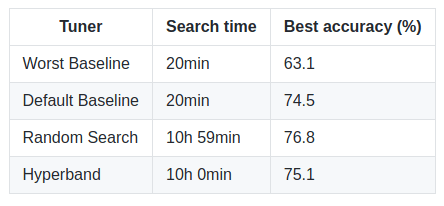# Hands on hyperparameter tuning with Keras Tuner

Rédigé par Julie ProstThis post will explain how to perform automatic hyperparameter tuning with Keras Tuner and Tensorflow 2.0 to boost accuracy on a computer vision problem.

Here you are : your model is running and producing a first set of results. However they fall far from the top results you were expecting. You're missing one crucial step : hyperparameter tuning!

In this post, we'll go through a whole hyperparameter tuning pipeline step by step. Full code is available on Github.

### What is hyperparameter tuning and why you should care

A machine learning model has two types of parameters:

• trainable parameters, which are learned by the algorithm during training. For instance, the weights of a neural network are trainable parameters.
• hyperparameters, which need to be set before launching the learning process. The learning rate or the number of units in a dense layer are hyperparameters.

Hyperparameters can be numerous even for small models. Tuning them can be a real brain teaser but worth the challenge: a good hyperparameter combination can highly improve your model's performance. Here we'll see that on a simple CNN model, it can help you gain 10% accuracy on the test set!

Thankfully, open-source libraries are available to automatically perform this step for you!

### Tensorflow 2.0 and Keras Tuner

Tensorflow is a vastly used, open-source, machine learning library. In September 2019, Tensorflow 2.0 was released with major improvements, notably in user-friendliness. With this new version, Keras, a higher-level Python deep learning API, became Tensorflow's main API.

Shortly after, the Keras team released Keras Tuner, a library to easily perform hyperparameter tuning with Tensorflow 2.0. This post will show how to use it with an application to object classification. It will also include a comparison of the different hyperparameter tuning methods available in the library.

### Hyperparameter tuning with Keras Tuner

Before diving into the code, a bit of theory about Keras Tuner. How does it work?Hyperparameter tuning process with Keras Tuner

First, a tuner is defined. Its role is to determine which hyperparameter combinations should be tested. The library search function performs the iteration loop, which evaluates a certain number of hyperparameter combinations. Evaluation is performed by computing the trained model's accuracy on a held-out validation set.

Finally, the best hyperparameter combination in terms of validation accuracy can be tested on a held-out test set.

### Getting started

Let's get started! With this tutorial, you'll have an end-to-end pipeline to tune a simple convolutional network's hyperparameters for object classification on the CIFAR10 dataset.

#### Installation step

First, install Keras Tuner from your terminal:

``pip install keras-tuner``

You can now open your favorite IDE/text editor and start a Python script for the rest of the tutorial!

#### DatasetCIFAR10 random samples. The dataset is composed of 60000 images belonging to one out of 10 object classes.

This tutorial uses the CIFAR10 dataset. CIFAR10 is a common benchmarking dataset in computer vision. It contains 10 classes and is relatively small, with 60000 images. This size allows for a relatively short training time which we'll take advantage of to perform multiple hyperparameter tuning iterations.

Load and pre-process data:

The tuner expects floats as inputs, and the division by 255 is a data normalization step.

#### Model definition

Here, we'll experiment with a simple convolutional model to classify each image into one of the 10 available classes.Simple CNN representation, from this great blog post about CNNs

Each input image will go through two convolutional blocks (2 convolution layers followed by a pooling layer) and a dropout layer for regularization purposes. Finally, each output is flattened and goes through a dense layer that classify the image into one of the 10 classes.

In Keras, this model can be defined as below :

#### Search Space definition

To perform hyperparameter tuning, we need to define the search space, that is to say which hyperparameters need to be optimized and in what range. Here, for this relatively small model, there are already 6 hyperparameters that can be tuned:

• the dropout rate for the three dropout layers
• the number of filters for the convolutional layers
• the number of units for the dense layer
• its activation function

In Keras Tuner, hyperparameters have a type (possibilities are Float, Int, Boolean, and Choice) and a unique name. Then, a set of options to help guide the search need to be set:

• a minimal, a maximal and a default value for the Float and the Int types
• a set of possible values for the Choice type
• optionally, a sampling method within linear, log or reversed log. Setting this parameter allows to add prior knowledge you might have about the tuned parameter. We'll see in the next section how it can be used to tune the learning rate for instance
• optionally, a step value, i.e the minimal step between two hyperparameter values

For instance, to set the hyperparameter 'number of filters' you can use:

The dense layer has two hyperparameters, the number of units and the activation function:

Model Compilation

Then let's move to model compilation, where other hyperparameters are also present. The compilation step is where the optimizer along with the loss function and the metric are defined. Here, we'll use categorical entropy as a loss function and accuracy as a metric. For the optimizer, different options are available. We'll use the popular Adam:

Here, the learning rate, which represents how fast the learning algorithm progresses, is often an important hyperparameter. Usually, the learning rate is chosen on a log scale. This prior knowledge can be incorporated in the search through the setting of the sampling method:

#### Keras Tuner Hypermodels

To put the whole hyperparameter search space together and perform hyperparameter tuning, Keras Tuners uses `HyperModel` instances. Hypermodels are reusable class object introduced with the library, defined as follows:

The library already offers two on-the-shelf hypermodels for computer vision, HyperResNet and HyperXception.

#### Choose the tuner

Keras Tuner offers the main hyperparameter tuning methods: random search, Hyperband, and Bayesian optimization.

In this tutorial, we'll focus on random search and Hyperband. We won't go into theory, but if you want to know more about random search and Bayesian Optimization, I wrote a post about it: Bayesian optimization for hyperparameter tuning. As for Hyperband, its main idea is to optimize Random Search in terms of search time.

For every tuner, a seed parameter can be defined for experiments reproducibility: `SEED = 1`.

Random Search

The most intuitive way to perform hyperparameter tuning is to randomly sample hyperparameter combinations and test them out. This is exactly what the RandomSearch tuner does!

The objective is the function to optimize. The tuner infers if it is a maximization or a minimization problem based on its value.

Then, the `max_trials` variable represents the number of hyperparameter combinations that will be tested by the tuner, while the `execution_per_trial` variable is the number of models that should be built and fit for each trial for robustness purposes. The next section explains how to set them

Hyperband

Hyperband is an optimized version of random search which uses early-stopping to speed up the hyperparameter tuning process. The main idea is to fit a large number of models for a small number of epochs and to only continue training for the models achieving the highest accuracy on the validation set. The max_epochs variable is the max number of epochs that a model can be trained for.

#### Hyperparameters for the tuners?

You might be wondering how useful this whole process is seeing that several parameters also have to be set for the different tuners:

But here the problem is slightly different than the determination of hyperparameters. Indeed, these settings here will mostly depend on your computing time and resources. The highest number of trials you can perform, the better! Regarding the number of epochs, it's best if you know how many epochs your model needs to converge. You can also use early-stopping to prevent overfitting.

#### Hyperparameter tuning

Once the model and the tuner are set up, a summary of the task is easily available:

Tuning can start!

The search function takes as input the training data and a validation split to perform hyperparameter combinations evaluation. The epochs parameter is used in random search and Bayesian Optimization to define the number of training epochs for each hyperparameter combination.

Finally, the search results can be summarized and used as follows:

### Results

You can find this post code on Github. The following results were obtained after running it on an RTX 2080 GPU:Keras Tuner results. Worst baseline: model achieving the worst validation accuracy with one of random search's set of hyperparameters. Default baseline : obtained by setting all hyperparameters to their default value.

These results are far from the 99.3% accuracy achieved by state-of-the-art models on the CIFAR10 dataset but not so bad for such a simple network structure. You can already see notable improvement between the baselines and the tuned models, with a boost of more than 10% in accuracy between Random Search and the first baseline.

Overall, the Keras Tuner library is a nice and easy to learn option to perform hyperparameter tuning for your Keras and Tensorflow 2.O models. The main step you'll have to work on is adapting your model to fit the hypermodel format. Indeed, few standard hypermodels are available in the library for now.

Complementary documentation and tutorials are available on Keras Tuner’s website and their Github repo!

## Cet article à été écrit parJulie Prost